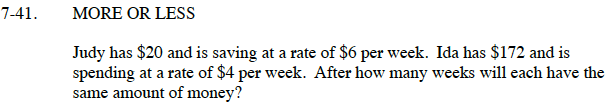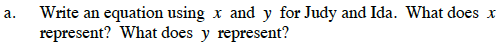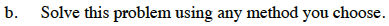### Home > CC3 > Chapter 7 > Lesson 7.2.1 > Problem7-41

7-41.
1. MORE OR LESS

2. Judy has $20 and is saving at a rate of$6 per week. Ida has $172 and is spending at a rate of$4 per week. After how many weeks will each have the same amount of money? Homework Help ✎

1. Write an equation using x and y for Judy and Ida. What does x represent? What does y represent?

2. Solve this problem using any method you choose.The equation for Judy is y = 6x + 20.
In this equation y represents the money she has and x represents the number of weeks that have passed.
Try to write your own equation for Ida.Try using the Equal Values Method.